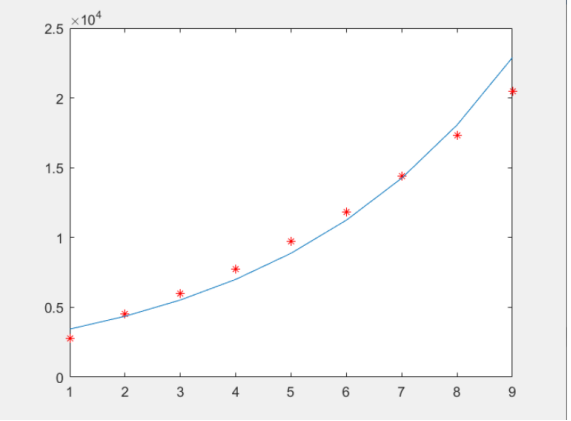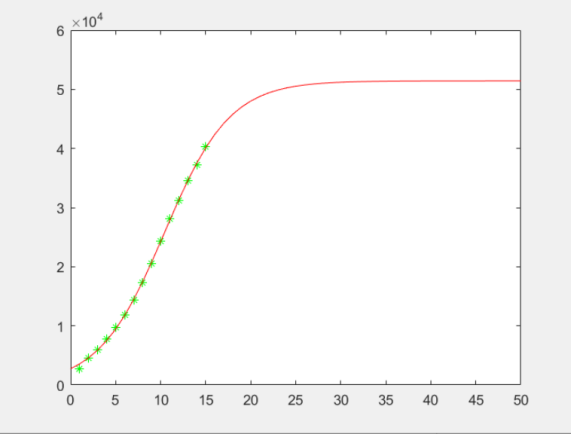# 人口增长模型

**人口增长模型我放假的时间（1.10）代入可预测到，一月十日就已经出现了上百名患者，当时对疫情的不重视导致了病毒传染的温床。我们可以看到，自1.26的七天之后，指数模型就已经出现了大的偏颇。x=0:10:1000y=0.02*(1-x/1000).*x;
grid on;
plot(x,y)

附录二：
x=dsolve('Dx=0.02*x*(1-x/1000)','x(0)=1','t')；
t=0:10:1000
x1=eval(x);
plot(t,x1,'k-')

附录三：
clear;
clc;
T= data(1:9,1);
X = data(1:9,2);
y=log(X);
t=[ones(9,1),T];%回归算法使用前加单一矩阵。
[b,bint,r,rint,stats]=regress(y,t);
rr=stats(1)%复相关系数
F=stats(2);%f统计量值
prob=stats(3)%概率xo=exp(b(1));
r=b(2);
py=xo*exp(r*T);
plot(T,X,'r*',T,py)%做对比图

附录四：
t= data(1:15,1);
x= data(1:15,2);
beta0=[50000 0.1];
[beta,r,J]=nlinfit(t,x,'volum1',beta0);
plot(t,x,'g*');
hold on;
yi=volum1(beta,t);
plot(t,yi,'r');

Volum1.m文件：
function  y1=volum1(beta,t)
y1=beta(1)./(1+((beta(1)./2761)-1).*exp(-beta(2)*t));©️2019 CSDN 皮肤主题: 数字20 设计师: CSDN官方博客